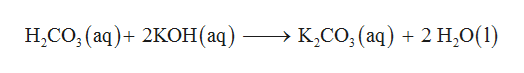I needed help figuring out how to write a net ionic equation for the overall reaction that occurs when aqueous solutions of carbonic acid and potassium hydroxide are combined. Assume excess base.I also would like you to say whether each part in the ionic equation is a solid, liquid, gas, or aqueous.

Question

I needed help figuring out how to write a net ionic equation for the overall reaction that occurs when aqueous solutions of carbonic acid and potassium hydroxide are combined. Assume excess base.

I also would like you to say whether each part in the ionic equation is a solid, liquid, gas, or aqueous.

Step 1

Carbonic acid (H2CO3) is a weak acid so only partial dissociation takes place whereas base potassium hydroxide (KOH) is...help_outlineImage TranscriptioncloseН.СО, (аq) + 2KОН (ад) —> К,со, (aq) + 2 Н,0(1) fullscreen

Want to see the full answer?

See Solution

Want to see this answer and more?

Our solutions are written by experts, many with advanced degrees, and available 24/7

See Solution
Tagged in

General Chemistry